Start typing, then use the up and down arrows to select an option from the list.# Partial Pressure

Jules Bruno
240views
1
So we know at this point that the total pressure felt within a container is a result of adding up. All the partial pressures of the gas is present. Now, if we can focus on one of these gasses and we know it's moles, its temperature and its volume, we could also find its partial pressure. Now we're going to stay here. If you assume that the gasses behave ideally, then their partial pressures can be calculated from the ideal gas law. We're gonna say here that the pressure of that gas that I'm focusing on so let's call a gas one. We confined its partial pressure if we know it's moles. So moles one. Ours are gas, constant times the temperature of the container divided by the volume of the container. So here we're using the ideal gas law to just focus in on one gas and from it determine its partial pressure.00:44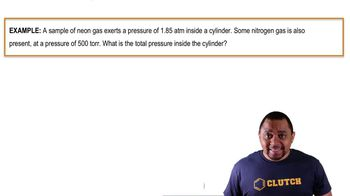02:09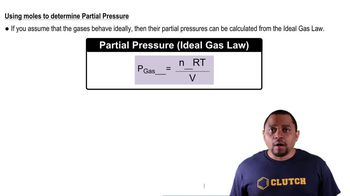00:48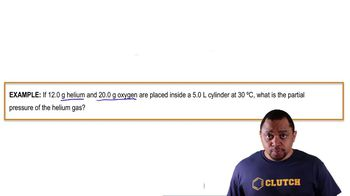03:26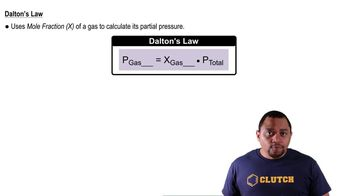00:27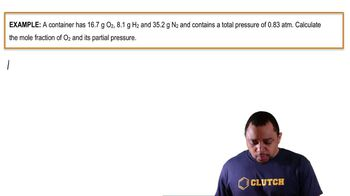03:18